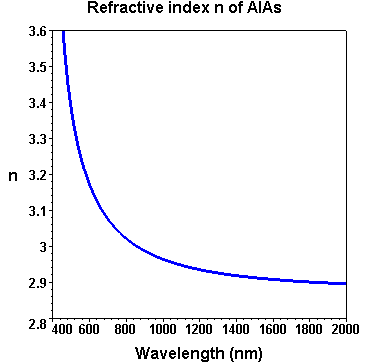> Energy band gap> GaAs | AlxGa1-xAs | InxGa1-xAs
> Refractive index > GaAs | AlAs | AlxGa1-xAs | InxGa1-xAs
> Devices > Bragg mirror | SAM | RSAM | SA | SANOS | SOC | Microchip | PCA
> Device application > Papers | Patents | FAQs## Refractive index n of AlAs

### Equation

According to Sadao Adachi J. Appl. Phys. Vol. 58, No.3, (1985) R1 the real part of the refractive index n of AlAs below the band gap edge can be expressed by: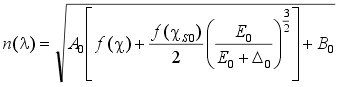with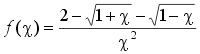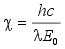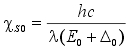In this equation the symbols and constants have the following meaning in the case of AlAs at room temperature:

• n - (real part) of the refractive index
• λ - vacuum wavelength
• h = 6.626 10 -34 Js - Planck´s constant
• c = 2.998 108 m/s - speed of light in vacuum
• hc/λ - photon energy
• A0 = 25.3 - fitted constant
• B0 = - 0.8 - fitted constant
• E0 = 2.95 eV - fundamental band gap at Γ-point
• E0 + Δ0 = 3.25 eV with Δ0 - spin-orbit splitting energy

### Numerical values

λ (nm) n(AlAs)
450 3.677
500 3.380
550 3.250
600 3.170
650 3.115
700 3.074
750 3.044
800 3.021
900 2.986
1000 2.963
1200 2.934
1500 2.912
2000 2.895